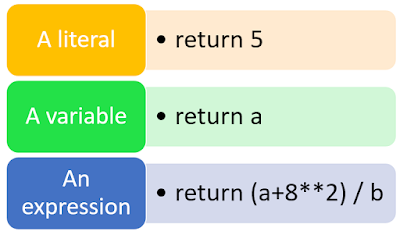In my Previous Post, I talked about the Introduction of functions, where we discussed how to create the first python function. At the time of the creation of the first python function, we used two important keywords def and return.

In this post, I am going to discuss those two important terms. read the article till the end and you will understand the importance of def and return keywords in Python.

After reading this article you will have a clear idea about the purpose of the def keyword in python and what does def do in python.

So, let's get started with the def keyword.

## def Statement

def is used to define a function. define means give the definition of the function. We start any function by def <Fun_Name> ( ), here function name is a valid identifier name and the two enclosing small brackets denote this is a function. Inside this two small parenthesis, you can provide arguments. Below are the important features of the function's def keyword:
• def executed at runtime (Remember, all we have in Python is runtime; there is no such thing as a separate compile time).
• The def statement is used to creates a function object with the name given after the def keyword. It is generally the function name we have provided.
• The definition of function happens at execution time. That means an object is created at runtime with the function name. Now as shown in the following diagram, we can assign a function name to a variable. That variable can call the function Now:

## Return Statement

return is used to return the computed result from the function to the caller of the function. the return keyword is generally used at the end of the function, but we can use this keyword at any suitable place in our python function. The syntax of writing the return keyword is return [<var>].  It is not compulsory to give the [<var>], we can omit return keyword also.

When we give the variable (var), the function returns the variable to the caller. If we do not give the return keyword the None will be returned to the caller.
• It returns values back to the caller.
• It is not compulsory to provide return statements in every function, OR we can use only return in place of return <variable>.
• If only return is used OR nothing is used it returns None.Return statement
A function can return literal, variable or any expression. If we return a literal the value will be returned directly. In the case of a variable, the variable's value will be returned and in the case of expression, the resultant value after calculation will be returned. You can understand the different values with the return keyword by the following image:

### Function can returnReturn Statement

### Returning more than one value:-

Using the return statement Programmer can return more than one value also. The programmer has to provide all the values in the comma-separated form. The values will be returned in the same order in which the Programmer writes them in the return statement. So, at the time of receiving the values at the place from where the function was called; the values should be received in the same order.

you can understand it with the following program. Here I am returning three literals 1, 2, 3 from the function fun(). I am receiving it in the same order a, b, c. So, the 1 will go into a, 2 will go in b, and 3 will go into c.

## Types of Functions

There are mainly three types of functions.
• Built-in Functions
• Ex. – print(), len()
• Functions defined in Modules
• Ex. – math module [ sin() ]
• User Defined Functions
• Ex.- we have seen DoSomething() in the previous Slide.

#### The flow of Execution in a Function Call

The order of execution of the statements at the runtime is called the flow of execution of a program run.

There are many advantages to the functions, some of the advantages are given below.
• Program development made easy and fast
• Program testing becomes easy
• Codesharing becomes possible
• Code re-usability increases